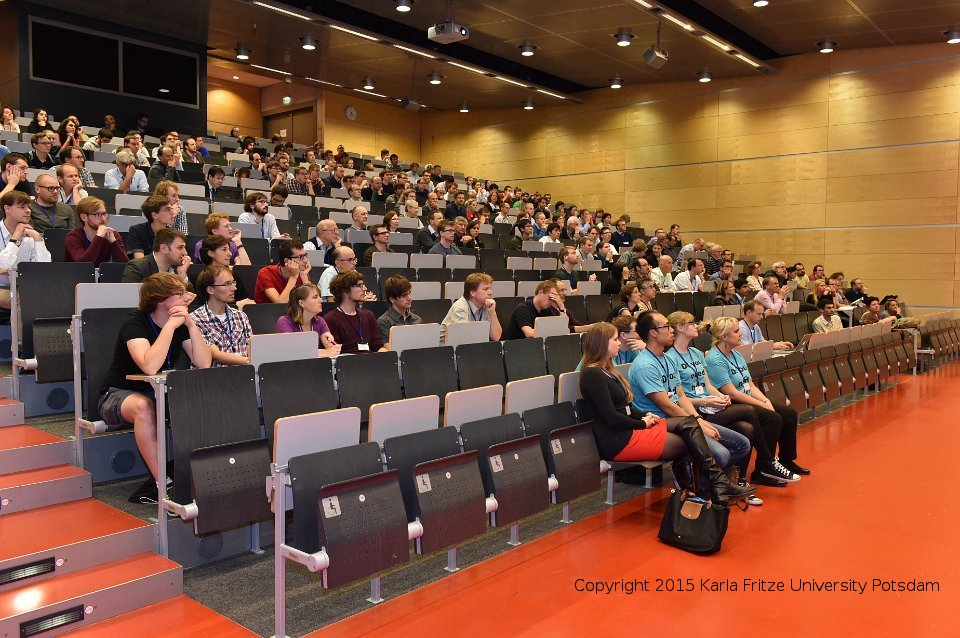## Prof. Raul Tempone will give a minisymposium talk at the International Conference on Scientific Computation And Differential Equations 2015 (SciCADE 2015), September 14-18, Potsdam, Germany

16 September, 2015

Multi-Index Monte Carlo

We propose and analyze a novel Multi-Index Monte Carlo (MIMC) method for weak approximation of stochastic models that are described in terms of differential equations either driven by random measures or with random coefficients. The MIMC method is both a stochastic version of the combination technique introduced by Zenger, Griebel and collaborators and an extension of the Multilevel Monte Carlo (MLMC) method first described by Heinrich and Giles. Inspired by Giles’s seminal work, we use in MIMC high-order mixed differences instead of using first-order differences as in MLMC to reduce the variance of the hierarchical differences dramatically. This in turn yields new and improved complexity results, which are natural generalizations of Giles’s MLMC analysis and which increase the domain of the problem parameters for which we achieve the optimal convergence, O(T OL−2 ). Moreover, in MIMC, the rate of increase of required memory with respect to TOL is independent of the number of directions up to a logarithmic term which allows far more accurate solutions to be calculated for higher dimensions than what is possible when using MLMC. We motivate the setting of MIMC by first focusing on a simple full tensor index set. We then propose a systematic construction of optimal sets of indices for MIMC based on properly defined profits that in turn depend on the average cost per sample and the corresponding weak error and variance. Under standard assumptions on the convergence rates of the weak error, variance and work per sample, the optimal index set turns out to be the total degree (TD) type. In some cases, using optimal index sets, MIMC achieves a better rate for the computational complexity than the corresponding rate when using full tensor index sets. We also show the asymptotic normality of the statistical error in the resulting MIMC estimator and justify in this way our error estimate, which allows both the required accuracy and the confidence level in our computational results to be prescribed. Finally, we include numerical experiments involving a partial differential equation posed in three spatial dimensions and with random coefficients to substantiate the analysis and illustrate the corresponding computational savings of MIMC.